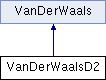JDFTx  1.7.0VanDerWaalsD2 Class Reference

DFT-D2 pair potential dispersion correction . More...

#include <VanDerWaalsD2.h>

Inheritance diagram for VanDerWaalsD2:## Classes

struct  AtomParams
C6 and R0 parameters for the VDW interactions. More...

## Public Member Functions

VanDerWaalsD2 (const Everything &e, string reason="")

virtual double getScaleFactor (string exCorrName, double scaleOverride=0.) const

virtual double energyAndGrad (std::vector< Atom > &atoms, const double scaleFac, matrix3<> *E_RRT=0) const

double energyAndGrad (const std::vector< std::vector< vector3<> > > &atpos, const ScalarFieldTildeArray &Ntilde, const std::vector< int > &atomicNumber, const double scaleFac, ScalarFieldTildeArray *grad_Ntilde=0, struct IonicGradient *forces=0, matrix3<> *E_RRT=0) const

AtomParams getParams (int atomicNumber, int sp) const
retrieve vdW parameters for an atomPublic Member Functions inherited from VanDerWaals
VanDerWaals (const Everything &e)

## Static Public Attributes

static const int unitParticle = -1
special atomic number used by some fluids: point particle with C6=1 J-nm^6/mol and R0=0Protected Attributes inherited from VanDerWaals
const Everythinge

## Detailed Description

DFT-D2 pair potential dispersion correction .

## Member Function Documentation

 double VanDerWaalsD2::energyAndGrad ( const std::vector< std::vector< vector3<> > > & atpos, const ScalarFieldTildeArray & Ntilde, const std::vector< int > & atomicNumber, const double scaleFac, ScalarFieldTildeArray * grad_Ntilde = 0, struct IonicGradient * forces = 0, matrix3<> * E_RRT = 0 ) const

Van der Waal correction to the interaction energy between the explicit atoms (from IonInfo) and the continuous fields Ntilde with specified atomic numbers. The gradient w.r.t site densities is accumulated to grad_Ntilde (if non-null), the negative gradient w.r.t discrete atom positions is accumulated to forces (if non-null) and the lattice gradient is accumulated to E_RRT (if non-null)

 virtual double VanDerWaalsD2::energyAndGrad ( std::vector< Atom > & atoms, const double scaleFac, matrix3<> * E_RRT = 0 ) const
virtual

Van der Waal correction energy for a collection of discrete atoms at fixed locations. Corresponding forces should be accumulated to Atom::force for each atom. If E_RRT is non-null, accumulate contributions to the symmetric lattice derivative (stress * volume).

Implements VanDerWaals.

## ◆ getScaleFactor()

 virtual double VanDerWaalsD2::getScaleFactor ( string exCorrName, double scaleOverride = 0. ) const
virtual

Retrieve the scale factor for a specified exchange-correlation functional. Use scaleOverride, if supplied and supported, to override the functional's default. Quit with an appropriate error message if functional is not parametrized.

Implements VanDerWaals.

The documentation for this class was generated from the following file:
• electronic/VanDerWaalsD2.h